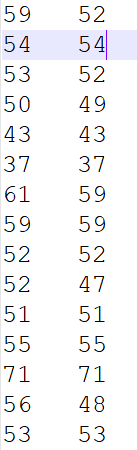pack python 二维数组_python socket变成 pack组包 - CSDN

• 代码如下所示： import struct class data_process(): def write_data(self,filename, data): fw = open(filename, "wb") for i in data: s = struct.pack('d', i) print('s...
代码如下所示：

import struct
class data_process():
def write_data(self,filename, data):
fw = open(filename, "wb")
for i in data:
s = struct.pack('d', i)
print('s',s)
fw.write(s)
fw.close()
fr = open(filename, 'rb')
print('unbytes',unbytes)
return unbytes
if __name__ == "__main__":
data_p = data_process()
a = [1, 2]
data_p.write_data('data', a)
data_p.read_data('data', a)

其中打包地时候的参数d表示double类型，更多类型参考以下博文：

https://www.cnblogs.com/qq78292959/archive/2013/04/07/3003607.html

展开全文• NumPy的ndarray数组对象不能像list一样动态地改变其大小，在做数据采集时很不方便。本文介绍如何通过np.frombuffer()...Python的列表对象实际上是一个动态指针数组。当列表中没有空间储存新的元素时，列表会动态地改变
本文转自：http://hyry.dip.jp/tech/slice/slice.html/13
NumPy的ndarray数组对象不能像list一样动态地改变其大小，在做数据采集时很不方便。本文介绍如何通过np.frombuffer()实现动态数组。

列表对象的内存动态分配

Python的列表对象实际上是一个动态指针数组。当列表中没有空间储存新的元素时，列表会动态地改变其大小，以容纳新的元素。每次改变大小时，它都会预留一部分空间，以降低改变大小的频率。下面的程序可以观察列表的这一行为。

import sys
import pylab as pl

size = []
for i in xrange(10000):
size.append(sys.getsizeof(size))

pl.plot(size, lw="2")
pl.show()

程序的输出如下图所示，图中每个阶梯跳变的位置都表示一次内存分配，而每个阶梯的高度表示额外分配的内存的大小。因此由于往列表中添加新元素时，基本上时间复杂度都为O(1)，只有在重新分配内存时，时间复杂度才变为O(n)。由于每次额外分配的内存和列表的长度成正比，因此随着列表的增大，重新分配内存的次数会减少，从而整体上append()方法的平均时间复杂度为O(1)。这种动态数组很适合用来做数据采集，然而由于列表中的每个元素都是对象，比较浪费内存，因此用列表做大量数据的采集并不划算。我们希望通过类似NumPy数组的对象采集数据。

NumPy数组的动态分配

NumPy的数组没有这种动态改变大小的功能，numpy.append()函数每次都会重新分配整个数组，并把原来的数组复制到新数组中。下面的程序模拟列表的动态分配，从而实现动态数组：

import numpy as np
class DynamicArray(object):
def __init__(self, item_type):
self._data = np.zeros(10, dtype=item_type)
self._size = 0

def get_data(self):
return self._data[:self._size]

def append(self, value):
if len(self._data) == self._size:
self._data = np.resize(self._data, int(len(self._data)*1.25))
self._data[self._size] = value
self._size += 1

item_type = np.dtype({
"names":["id", "x", "y", "z"],
"formats":["i4", "f8", "f8", "f8"]})

da = DynamicArray(item_type)

for i in xrange(100):
da.append((i, i*0.1, i*0.2, i*0.3))

data = da.get_data()

用array数组采集数据

Python标准库中的array数组也提供了动态分配内存的功能，而且它和NumPy数组一样直接将数值的二进制数据保存在一块内存中，因此我们可以先用array数组收集数组，然后通过np.frombuffer()将array数组的数据内存直接转换为一个NumPy数组。下面是一个例子：

>>> import numpy as np
>>> from array import array
>>> a = array("d", [1,2,3,4])   # 创建一个array数组
>>> a
array('d', [1.0, 2.0, 3.0, 4.0])
>>> na = np.frombuffer(a, dtype=np.float) # 通过np.frombuffer()创建一个和a共享内存的NumPy数组
>>> na
array([ 1.,  2.,  3.,  4.])
>>> na = 20  # 修改NumPy数组中的第一个元素
>>> a
array('d', [1.0, 20.0, 3.0, 4.0])  # array数组中的第一个元素也同时改变

array数组只支持一维，如果我们需要采集多个频道的数据，可以将这些数据依次添加进array数组，然后通过reshape()方法将np.frombuffer()所创建的NumPy数组改为二维数组。下面是一个例子：

buf = array("d")
for i in range(100):
buf.append(math.sin(i*0.1)) ❶
buf.append(math.cos(i*0.1))

data = np.frombuffer(buf, dtype=np.float).reshape(-1, 2) ❷
print data

在这个例子中，❶我们通过array数组buf采集两个频道的数据，数据采集完毕之后，我们通过np.frombuffer()将其转换为NumPy数组，并通过reshape()将其形状改为(100,2)。

用bytearray采集数据

当每个频道的数据类型不同时，就不能采用上节所介绍的方法了。这时我们可以使用bytearray收集数据。bytearray是字节数组，因此我们首先需要通过struct模块将Python的数值转换成其字节表示形式。如果数据来自二进制文件或者硬件，那么我们得到得已经是字节数据，这个步骤可以省略。下面是使用bytearray进行数据采集的例子：

buf = bytearray()
for i in range(100):
buf.extend(struct.pack("=hdd", i, math.sin(i*0.1), math.cos(i*0.1))) ❶

dtype = np.dtype({"names":["id","sin","cos"], "formats":["h", "d", "d"]}) ❷
data = np.frombuffer(buf, dtype=dtype) ❸
print data

❶采集三个频道的数据，其中频道1是短整型整数，其类型符号为”h”，频道2和3为双精度浮点数，其类型符号为”d”。类型格式字符串中的”=”表示输出得字节数据不进行内存对齐。即一条数据的字节数为2+8+8=16，如果没有”=”，那么一条数据的字节数则为8+8+8=24。

❷定义一个dtype对象表示一条数据的结构，dtype对象缺省不进行内存对齐，如果采集数据用的bytearray中的数据是内存对齐的话，只需要设置dtype()的align参数为True即可。

❸最后通过np.frombuffer()将bytearray转换为NumPy的结构数组。然后我们就可以通过data[“id”]、data[“sin”]和data[“cos”]访问三个频道的数据了。

np.frombuffer()还可以从字符串创建数组，数组也和字符串共享数据内存，但由于字符串是不可变对象，因此所创建的数组是只读的。如果不需要修改数据，这种方法比np.fromstring()更快、更节省内存。


展开全文• 我需要把一个2*50000的数据保存到...写入数据 acc_array是一个2*50000的二维数组 f=open('PATH/test.txt','w') for j in range(50000): f.write(str(acc_array[j])) f.write(' ') f.write(str(acc_array[j...
  我需要把一个2*50000的数据保存到本地，然后再从其中读取1.写入数据  acc_array是一个2*50000的二维数组 f=open('PATH/test.txt','w')
for j in range(50000):
f.write(str(acc_array[j]))
f.write('   ')
f.write(str(acc_array[j])+'\n')
f.close()这里的换行保存，打开记事本文件的时候发现并没有换行，没关系，以word或者notepad打开都是换行的 而且不影响读取保存数据截图2.读取数据,按照行读取我这里是把每一行的两个数字读取出来，相除后产生一个新数组import numpy as np
file=open('test.txt','r')
#a=[*2 for i in range(50000)]
a=[]
m=''
n=''
for j in range(50000):
print(line)
for i in range(len(line)):
if line[i]>='0' and line[i]<='9':
m=m+line[i]
else:
break
for t in range(i,len(line)):
if line[t]>='0' and line[t]<='9':
n=n+line[t]
print(m,'*******',n)
a.append(float((1.0*int(n))/(1.0*int(m))))
print(a[j])
m=''
n=''
if not line:
break

print(line)

print(np.argsort(a))3.写入byte数据我想写入一串字节数据‘0110101101110001010100100’到文件，可以用以下方式(需要import struct)    savedBinFile = open('/home/ximao/test.bin', "wb");  # open a file, if not exist, create it
for i in range(len(bt) // 8):
if bt[8 * i] == '0':
savedBinFile.write(struct.pack('b', int(bt[i * 8 + 1:(i + 1) * 8], 2)));
else:
savedBinFile.write(struct.pack('b', -int(bt[i * 8 + 1:(i + 1) * 8], 2)));
for i in range(len(wb) // 8):
if wb[8 * i] == '0':
savedBinFile.write(struct.pack('b', int(wb[i * 8 + 1:(i + 1) * 8], 2)));
else:
savedBinFile.write(struct.pack('b', -int(wb[i * 8 + 1:(i + 1) * 8], 2)));
savedBinFile.close()这里每次写入8位bit数据。即一个字节，之所以判断第一位是否为0是因为一个字节能表示的数位[-128，127]，第一位是符号为，但是如果我们每次int(bt[i * 8 :(i + 1) * 8], 2)),这样8bit数据表示的整数实际上会超过[-128,127]，所以我们取后面七位，然后再在前面加入正负号即可
展开全文python
• 本文本打算写toimage这个函数的，发现网上有很多将数组保存为图像的方法，故一起整理在一起。其他方法是参考了：Numpy数组保存为图片Numpy数组类型的矩阵，如何将它作为图像写入磁盘？任何格式的图像都行(PNG，JPEG...
本文本打算写toimage这个函数的，发现网上有很多将数组保存为图像的方法，故一起整理在一起。其他方法是参考了：Numpy数组保存为图片Numpy数组类型的矩阵，如何将它作为图像写入磁盘？任何格式的图像都行(PNG，JPEG，BMP ...)最佳的解决方法可以使用scipy.misc,代码如下：import scipy.misc
scipy.misc.imsave('outfile.jpg', image_array)这里顺带说下scipy.misc.toimage函数函数原来形式如下：scipy.misc.toimage(*args,**kwds)这个函数将在scipy 1.2.0版本中删除，将会用Pillow的Image.formarray替代。*args：输入的array;**kwds：输入的关键字；功能是输入一个numpy数组array,输出一个PIL图像。这个功能只有在PIL安装后才能使用。输出的PIL图的模式依赖于array的形状和pal, mode这些关键字。对于2-D arrays, 如果pal 是有效的（N，3） byte类的且其值在（0，255）间的RGB值, 那么mode='P'，否则mode='L'， 除非mode给出为'F'或‘I’，这种情况下将会创建浮点数 和/或 整数数组。对于3-D arrays, ‘channel_axis’参数指示数组的哪个维度会保存通道数据。对于3-D arrays, 如果某个维度是3， 默认mode是RGB或YCbCr。numpy array 必须是2维或3维的。函数可写成scipy.misc.toimage（ARR，高=255，低= 0，的Cmin =无，Cmax为无，PAL =无，模式=无，channel_axis =无）上面的scipy版本会标准化所有图像，以便min(数据)变成黑色，max(数据)变成白色。如果数据应该是精确的灰度级或准确的RGB通道，则解决方案为：
import scipy.miscscipy.misc.toimage(image_array, cmin=0.0, cmax=...).save('outfile.jpg')（SciPy 中包含一些用于输入和输出的实用模块。下面介绍其中两个模块：io 和misc。以图像形式保存数组因为我们需要对图像进行操作，并且需要使用数组对象来做运算，所以将数组直接保存为图像文件非常有用。imsave() 函数可以从scipy.misc 模块中载入。要将数组im 保存到文件中，可以使用下面的命令：from scipy.misc import imsave  imsave('test.jpg',im) ）我在程序中的一个实例是这样的：for i in range(20):
image_array=mnist.train.images[i,:]
image_array=image_array.reshape(28,28)
filename=save_dir+'mnist_train_%d.jpg' % i
scipy.misc.toimage(image_array,cmin=0.0,cmax=1.0).save(filename)第二种解决办法使用PIL。给定一个numpy数组"A"：from PIL import Imageim = Image.fromarray(A)im.save("your_file.jpeg")你可以用几乎任何你想要的格式来替换"jpeg"。有关格式详见here更多细节第三种办法纯Python(2& 3)，没有第三方依赖关系的代码片段。此函数写入压缩的真彩色(每个像素4个字节)RGBA PNG。def write_png(buf, width, height):    """ buf: must be bytes or a bytearray in Python3.x,        a regular string in Python2.x.    """    import zlib, struct     # reverse the vertical line order and add null bytes at the start    width_byte_4 = width * 4    raw_data = b''.join(b'\x00' + buf[span:span + width_byte_4]                        for span in range((height - 1) * width_byte_4, -1, - width_byte_4))     def png_pack(png_tag, data):        chunk_head = png_tag + data        return (struct.pack("!I", len(data)) +                chunk_head +                struct.pack("!I", 0xFFFFFFFF & zlib.crc32(chunk_head)))     return b''.join([        b'\x89PNG\r\n\x1a\n',        png_pack(b'IHDR', struct.pack("!2I5B", width, height, 8, 6, 0, 0, 0)),        png_pack(b'IDAT', zlib.compress(raw_data, 9)),        png_pack(b'IEND', b'')])...数据应直接写入以二进制打开的文件，如下所示：data = write_png(buf, 64, 64)with open("my_image.png", 'wb') as fd:    fd.write(data)Original source另见：Rust Port from this question.使用示例感谢@Evgeni Sergeev：https://stackoverflow.com/a/21034111/432509第四种办法用matplotlib：import matplotlib matplotlib.image.imsave('name.png', array)适用于matplotlib 1.3.1，不确定更低的版本是否有效。文档：Arguments:  *fname*:    A string containing a path to a filename, or a Python file-like object.    If *format* is *None* and *fname* is a string, the output    format is deduced from the extension of the filename.  *arr*:    An MxN (luminance), MxNx3 (RGB) or MxNx4 (RGBA) array.第五种办法如果使用matplotlib，也可以这样做：import matplotlib.pyplot as pltplt.imshow(matrix) #Needs to be in row,col orderplt.savefig(filename)这将保存plot(而不是图像本身)。第6种办法python的opencv(http://docs.opencv.org/trunk/doc/py_tutorials/py_tutorials.html)。import cv2import numpy as np cv2.imwrite("filename.png", np.zeros((10,10)))如果你需要做更多的处理，而不是保存，这个库比较有用。参考文献Saving a Numpy array as an image
展开全文write PIL
• 有一个Numpy数组类型的矩阵，如何将它作为图像写入磁盘？任何格式的图像都行(PNG，JPEG，BMP ...)。 最佳解决办法 可以使用scipy.misc，代码如下： import scipy.misc scipy.misc.imsave('outfile.jpg', ...python 图像 数组
• ## struct.pack用法

万次阅读 2017-12-05 16:55:50
struct.pack用于将Python的值根据格式符，转换为字符串（因为Python中没有字节(Byte)类型，可以把这里的字符串理解为字节流，或字节数组）。其函数原型为：struct.pack(fmt, v1, v2, …)，参数fmt是格式字符串，关于...
• import struct with open(file_name_dir, 'wb')as fp: for x in data_pack: a = struct.pack('i', int(x)) fp.write(a) fp.close() pack的格式：Python 写入 二进制 文件
• Python的列表对象实际上是一个动态指针数组。当列表中没有空间储存新的元素时，列表会动态地改变其大小，以容纳新的元素。每次改变大小时，它都会预留一部分空间，以降低改变大小的频率。下面的程序可以观察列表的这...python
• 文档英文原版参见NDArray - Imperative tensor operations on CPU/GPU ...如果你对python的科学计算包Numpy熟悉的话，你会发现mxnet.ndarray与numpy.ndarray在诸多方面十分相似。就像对应的NumPy数据结构，M深度学习框架 文档
• ## 最全ctypes用法总结

万次阅读 多人点赞 2018-08-22 15:51:31
ctypes是Python的一个外部库，提供和C语言兼容的数据类型，可以很方便地调用DLL中输出的C接口函数。 #### 1.加载dll和取出函数 `python from ctypes import * dll = cdll.LoadLibrary(dllpath) #dllpath是字符串...
• 注：我使用的环境：IDLE (Python 3.6 32-bit) ；vs2010 以下环境配置部分详见：混合编程之——C++调用python2.7&amp;python3.5 以python3.6为例： 需要单独将Python36-32下面的，include文件夹里面的头文件和...
• 1. 0，1问题 ...我们可以一步一步来 ，先创建一个表格 (数组), 数组dp[i][j] i代表你只用前i个物体，j代表你的剩余容量，得到一个表格 先填第一行(也就是假设现在你只有第一个物体), 首...背包问题 动态规划 数据结构与算法
• 如果你对python的科学计算包Numpy熟悉的话，你会发现mxnet.ndarray与numpy.ndarray在诸多方面十分相似。源网址：CPU/GPU Array Manipulation   基础 多维数组是一组同类型数据的集合，例如一个3D空间中的点的...python 深度学习
• ## np.frombuffer()

千次阅读 2019-07-13 11:56:06
numpy.frombuffer numpy.frombuffer(buffer,dtype=float,count=-1,offset=0) Interpret a buffer as a 1-dimensional array. Parameters: buffer:buffer_like ...An object that exposes the buffer inte...
• ## C语言简答题汇总

千次阅读 2020-05-07 16:08:36
1、c语言的特点以及C语言与Python的比较？ c语言的数据类型丰富，具有现代语言的各种数据结构，能实现复杂的运算。c语言使，用灵活方便，便于实现程序的结构化，节省内存。 C语言与Python的比较： （1）首先Python的...
• 2.二维数组这样定义可以修改固定位置的值： rawDataArray_temp = [(*nIRImageWidth)for i in range(nIRImageHight)] rawDataArray_temp[j][i] = 123 #i行j列 3.数据转byte使用st...
• 0-1背包问题是最简单的问题，此外还要完全背包问题、多重背包问题、混合背包问题、二维费用背包问题、分组背包问题等等。好的参考资料可以见《背包问题九讲》：https://www.kancloud.cn/kancloud/pack/70124 其中0-...python 背包问题 动态规划
• Traceback (most recent call last): File "./vec.py", line 56, in pickle.dump(trainX, f, pickle.HIGHEST_PROTOCOL) File "/usr/lib/python2.7/pickle.py", line 1378, in dump Pickler(file, protoTensorflow struct 报错
• 2020最新Python零基础到精通资料教材，干货分享，新基础Python教材，看这里，这里有你想要的所有资源哦，最强笔记，教你怎么入门提升！让你对自己更加有信心，重点是资料都是免费的，免费！！！ 本视频是面向编程零...python 人工智能 编程语言 机器学习 java
• 简简单单讲map（一）map函数map BLOCK LISTmap EXPR, LISTmap函数对LIST里的每个元素按BLOCK或EXPR进行计算，遍历LIST时，临时将LIST里的每个元素赋值给\$_变量。map对每次的计算返回一个结果列表，它在列表上下文里...perl matrix dictionary filenames cgi
...